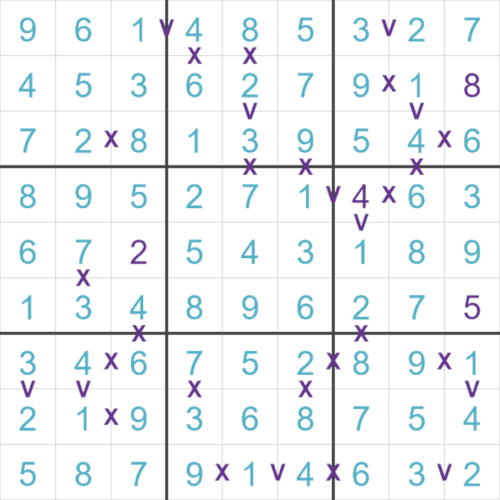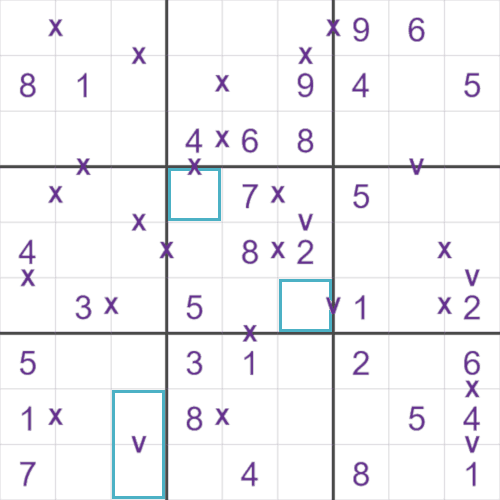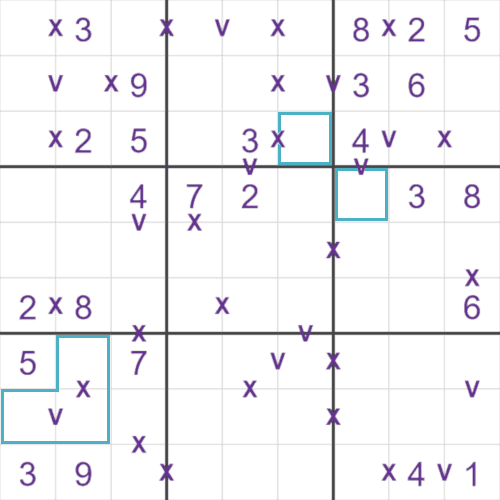# Center Dot Sudoku Rules

### A brief guideThis is an example of a completed SudokuXV grid. A SudokuXV puzzle uses the same base rules of Sudoku, but in addition some cells will have a 'V' or a 'X' between them. In the case of a 'V', these two cells will add to 5, and in the case of a 'X', these two cells will add to 10.
If you look at this puzzle, you can see that these rules are satisfied.This is an example SudokuXV starting grid, and I have highlighted some cells that we can either fill in, or insert some pencil marks. In the topmost cell, it is linked to a '4' by a 'X' symbol. The 'X' tells us that these two cells must add to 10, so the highlighted cell must be a '6'.
Slightly lower we have another highlighted cell linked by a 'V' to a 1. These two cells must add to 5, so the highlighted cell must be a 4.
At the bottom of the puzzle we have two highlighted cells linked with a 'V'. We know these two cells must add to 5, and there's only two ways we can do that, either 1+4=5, or 2+3=5. Because of the 1s and 4s already in these two rows we know that we can't have the combination of 1 and 4 in these cells. We can then fill in the pencil marks of 2 and 3 for these two cells.This is another starting SudokuXV grid, can you fill in the highlighted cells? The set of 3 cells in the bottom right can be filled (i.e. not with pencil marks), hint: Consider the 'V' clue first, and then consider what possibilities that would leave for the 'X' clue.